Next: Volume Integrals Up: Vectors and Vector Fields Previous: Surface Integrals

# Vector Surface Integrals

Surface integrals often occur during vector analysis. For instance, the rate of flow of a liquid of velocitythrough an infinitesimal surface of vector area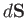is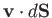. The net rate of flow through a surfacemade up of very many infinitesimal surfaces is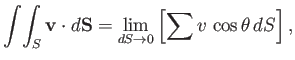(A.95)

whereis the angle subtended between the normal to the surface and the flow velocity.

Analogously to line integrals, most surface integrals depend both on the surface and the rim. But some (very important) integrals depend only on the rim, and not on the nature of the surface which spans it. As an example of this, consider incompressible fluid flow between two surfaces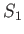andthat end on the same rim. (See Figure A.23.) The volume between the surfaces is constant, so what goes in must come out, and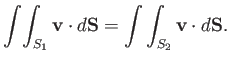(A.96)

It follows that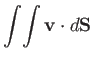(A.97)

depends only on the rim, and not on the form of surfacesand.Next: Volume Integrals Up: Vectors and Vector Fields Previous: Surface Integrals
Richard Fitzpatrick 2016-03-31